NEET  >  Energy Stored in a Capacitor

# Energy Stored in a Capacitor Notes | Study Physics Class 12 - NEET

## Document Description: Energy Stored in a Capacitor for NEET 2022 is part of Physics Class 12 preparation. The notes and questions for Energy Stored in a Capacitor have been prepared according to the NEET exam syllabus. Information about Energy Stored in a Capacitor covers topics like and Energy Stored in a Capacitor Example, for NEET 2022 Exam. Find important definitions, questions, notes, meanings, examples, exercises and tests below for Energy Stored in a Capacitor.

Introduction of Energy Stored in a Capacitor in English is available as part of our Physics Class 12 for NEET & Energy Stored in a Capacitor in Hindi for Physics Class 12 course. Download more important topics related with notes, lectures and mock test series for NEET Exam by signing up for free. NEET: Energy Stored in a Capacitor Notes | Study Physics Class 12 - NEET
 1 Crore+ students have signed up on EduRev. Have you?

Energy Stored in a Charged capacitor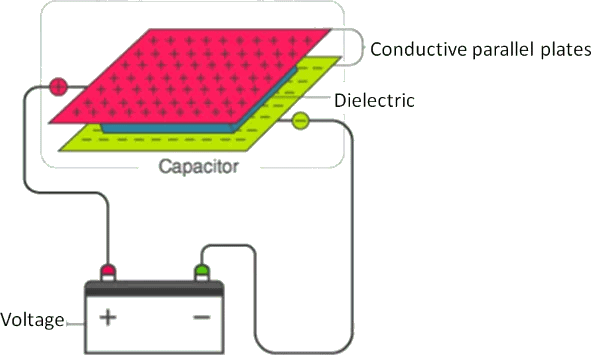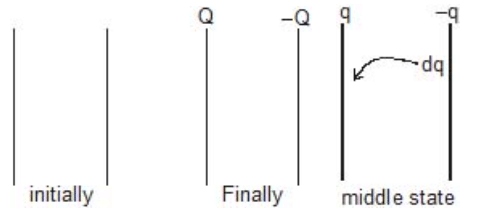Work has to be done in charging a conductor against the force of repulsion by the already existing charges on it. The work is stored as a potential energy in the electric field of the conductor. Suppose a conductor of capacity C is charged to a potential V0 and let q0 be the charge on the conductor at this instant. The potential of the conductor when (during charging) the charge on it was q (< q0) is,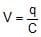Now, work done in bringing a small charge dq at this potential is,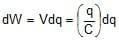Therefore, total work done in charging it from 0 to q0 is,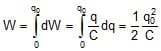This work is stored as the potential energy,

Therefore,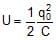Further by using q0 = CV0 we can write this expression also as,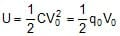In general if a conductor of capacity C is charged to a potential V by giving it a charge q, then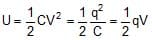3.1 Energy Density of a Charged Capacitor

This energy is localized on the charges or the plates but is distributed in the field. Since in case of a parallel plate capacitor, the electric field is only between the plates, i.e., in a volume (A × d), the energy density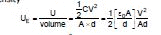or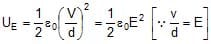3.2 Calculation of Capacitance

The method for the calculation of capacitance involves integration of the electric field between two conductors or the plates which are just equipotential surfaces to obtain the potential difference Vab. Thus,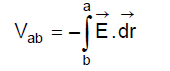Therefore,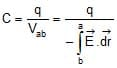3.3 Heat Generated :

(1) Work done by battery

W = QV

Q = charge flow in the battery

V = EMF of battery

(2) W = Ve (When Battery discharging)

W = -Ve (When Battery charging)

(3)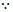Q = CV (C = equivalent capacitance)

so W = CV × V = CV2

Now energy on the capacitor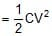Therefore, Energy dissipated in form of heat (due to resistance)

H = Work done by battery - {final energy of capacitor - initial energy of capacitor}

Ex.3 At any time S1 switch is opened and S2 is closed then find out heat generated in circuit.

Sol.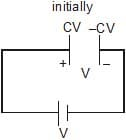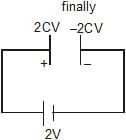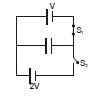Charge flow through battery = Qf - Qi

= 2CV - CV = CV

H = (CV × 2V) -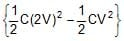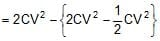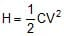Ex.4 (a) Find the final charge on each capacitor if they are connected as shown in the figure.

Sol. Initially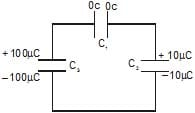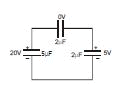Finally let q charge flows clockwise then

Now applying KVL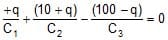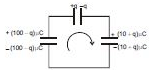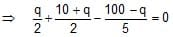5q + 50 + 5q - 200 + 2q = 0

12 q - 150 = 0 ⇒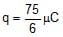so finally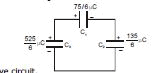(b) Find heat loss in the above circuit.

ΔH = Energy [initially - finally] on capacitor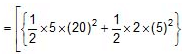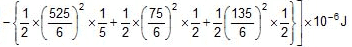Distribution of Charges on Connecting two Charged Capacitors :

When two capacitors C1 and C2 are connected as shown in figure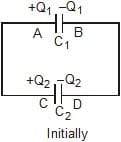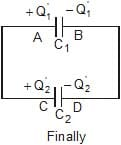Before connecting the capacitors Parameter Ist Capacitor IInd Capacitor Capacitance C1 C2 Charge Q1 Q2 Potential V1 V2

 After connecting the capacitors Parameter Ist Capacitor IInd Capacitor Capacitance C1 C2 Charge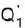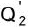Potential V1 V2

(a) Common potential :

By charge conservation on plates A and C before and after connection.

Q1 +  Q2 = C1V  + C2V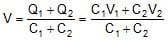=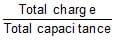(b)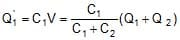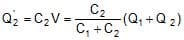(c) Heat loss during redistribution :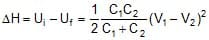The loss of energy is in the form of Joule heating in the wire.

• When plates of similar charges are connected with each other ( with and - with -) then put all values (Q1, Q2, V1, V2) with positive sign.
• When plates of opposite polarity are connected with each other ( with -) then take charge and potential of one of the plate to be negative.

Derivation of above formulae :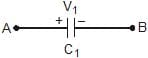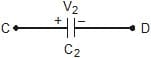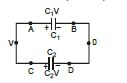Let potential of B and D is zero and common potential on capacitors is V, then at A and C it will be V.

C1V +  C2V = C1V1 + C2V2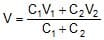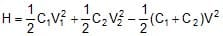=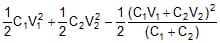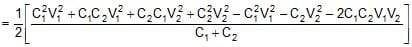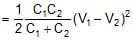H =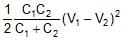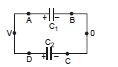when oppositely charged terminals are connected then

Therefore, C1V C2V = C1V1 - C2V2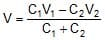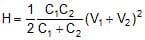Ex.5 Find out the following if A is connected with C and B is connected with D.

(i) How much charge flows in the circuit.

(ii) How much heat is produced in the circuit.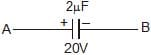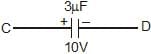Sol. Let potential of B and D is zero and common potential on capacitors is V, then at A and C it will be V.

By charge conservation,

3V + 2V = 40 + 30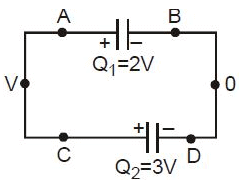5V = 70 ⇒ V = 14 volt

Charge flow = 40 - 28 = 12 μC

Now final charges on each plate is shown in the figure.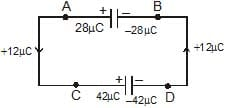(ii) Heat produced =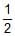× 2 × (20)2× 3 × (10)2 -× 5 × (14)2

= 400 150 - 490

= 550- 490 = 60 mJ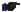• When capacitor plates are joined then the charge remains conserved.
• We can also use direct formula of redistribution as given above.

Ex.6 Repeat above question if A is connected with D and B is connected with C.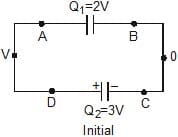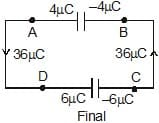Sol. Let potential of B and C is zero and common potential

on capacitors is V, then at A and D it will be V

2V +   3V = 10 ⇒ V = 2 volt

Now charge on each plate is shown in the figure.

Heat produced = 400 + 150 - 1

Therefore 2 × 5 × 4

= 550 - 10 = 540 μJ• Here heat produced is more. Think why ?

Ex.7 Three capacitors as shown of capacitance 1mF, 2mF and 2mF are charged upto potential difference 30 V, 10 V and 15V respectively. If terminal A is connected with D, C is connected with E and F is connected with B. Then find out charge flow in the circuit and find the final charges on capacitors.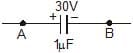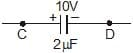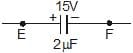Sol. Let charge flow is q.

Now applying Kirchhoff's voltage low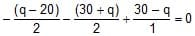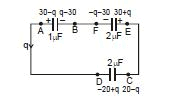- 2q = - 25

q = 12.5 mC

Final charges on plates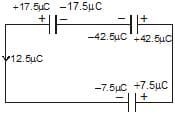The document Energy Stored in a Capacitor Notes | Study Physics Class 12 - NEET is a part of the NEET Course Physics Class 12.
All you need of NEET at this link: NEET

## Physics Class 12

157 videos|425 docs|213 tests
 Use Code STAYHOME200 and get INR 200 additional OFF

## Physics Class 12

157 videos|425 docs|213 tests

### How to Prepare for NEET

Read our guide to prepare for NEET which is created by Toppers & the best Teachers

Track your progress, build streaks, highlight & save important lessons and more!

,

,

,

,

,

,

,

,

,

,

,

,

,

,

,

,

,

,

,

,

,

;